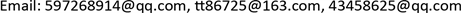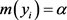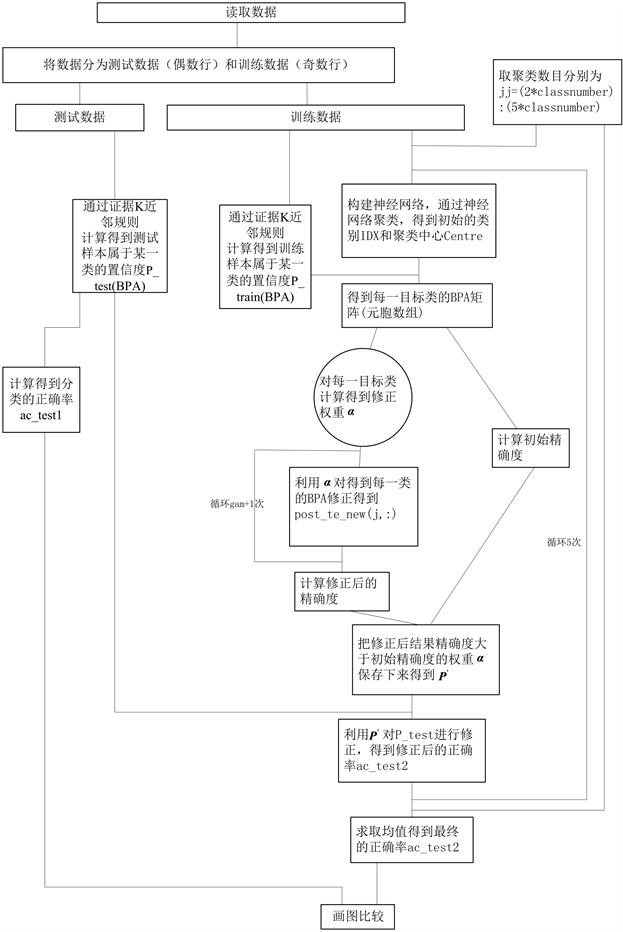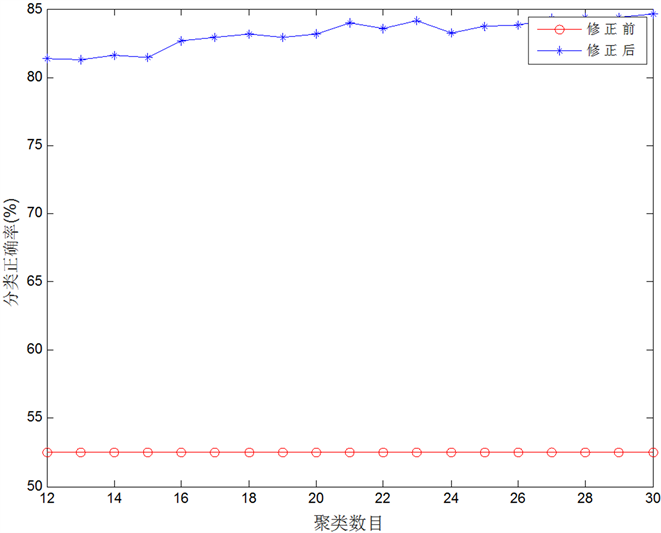﻿ 基于证据K近邻的目标识别新方法 A New Target Recognition Method Based on Evidence Theoretic K-NN Rule

Artificial Intelligence and Robotics Research
Vol. 08  No. 02 ( 2019 ), Article ID: 29183 , 9 pages
10.12677/AIRR.2019.82005

A New Target Recognition Method Based on Evidence Theoretic K-NN Rule

Xin Guan, Jing Zhao, Haiqiao Liu

Naval Aeronautical University, Yantai ShandongReceived: Feb. 6th, 2019; accepted: Mar. 1st. 2019; published: Mar. 8th, 2019ABSTRACT

This paper presents a new target recognition method based on evidence theoretic K-NN rule. A training correction step was added on the basis of Zouhal’s improved KNN algorithm. Firstly, a reference nearest neighbor distance of every target class should be computed in order to separate samples of one class from other samples with least error rate. Secondly, the initial classification result can be got through the reference nearest neighbor distance and DS evidence theory. Thirdly, setting up confusion matrix P and optimizing iteration through neural network to obtain matrix parameters for Zouhal’s classification result correction. Finally, the generalization ability of the matrix P is verified through multiple data sets, and the feasibility and effectiveness of the new method are verified by comparing with the classification accuracy of the classical algorithm.

Keywords:Evidence Theoretic K-NN Rule, Confusion Matrix, Target Recognition1. 引言

2. K近邻算法

K近邻(K-Nearest Neighbor, KNN)算法是在1968年由Cover和Hart提出来的  ，它是一种理论比较成熟的分类算法，也是最简单的机器学习算法之一。

2.1. 算法思路及特点

2.2. 算法描述

${d}_{ij}=\sqrt{\underset{l=1}{\overset{n}{\sum }}{\left({x}_{i}^{\left(l\right)}-{x}_{j}^{\left(l\right)}\right)}^{2}}$ (1)

$y=\mathrm{arg}\underset{{c}_{j}}{\mathrm{max}}\underset{{x}_{i}\in {N}_{K}\left(X\right)}{\sum }I\left({y}_{i}={c}_{j}\right)$ (2)

3. 证据K近邻规则

K近邻算法的一个显而易见的改进是对K个近邻的贡献进行加权  ，根据它们相对待识别样本 $X$ 的距离，将较大的权值赋给较近的近邻，因此，引入证据理论中，基本概率赋值函数的规律应该随距离的增大而减小，这里取负指数函数来表达  ，即

$\alpha ={\alpha }_{0}{\text{e}}^{-{d}_{k}}$ (3)

$y=\mathrm{arg}\underset{{c}_{j}}{\mathrm{max}}\underset{{x}_{i}\in {N}_{K}\left(X\right)}{\sum }\alpha I\left({y}_{i}={c}_{j}\right)$ (4)$\alpha \in \left[0,1\right]$ (5)

4. 新算法

4.1. 新算法的思想

4.2. 混淆矩阵P的定义与求解

$P=\left[\begin{array}{cccc}{P}_{11}& {P}_{12}& \cdots & {P}_{1j}\\ P{}_{21}& {P}_{22}& \cdots & {P}_{2j}\\ ⋮& ⋮& \ddots & ⋮\\ {P}_{i1}& {P}_{i2}& \cdots & {P}_{ij}\end{array}\right]$ (6)

$\alpha =\left[{\alpha }_{1},{\alpha }_{2},\cdots ,{\alpha }_{C}\right]$ (7)

$\underset{j=1}{\overset{N}{\sum }}{d}_{j}=‖\alpha P-T‖$ (8)

4.3. 新算法的步骤

①选取数据集样本进行训练，得出初始目标类和聚类中心；

②利用证据K近邻规则计算待测样本的初始分类结果；

③由式(8)最小化算得混淆矩阵 $P$

④利用混淆矩阵 $P$ 对结果进行修正，得出最终分类结果 $m\left({y}_{i}\right)=\alpha P$ ，其中 $i=1,2,\cdots ,C$

5. 实验仿真与分析

5.1. 仿真步骤

Satimage数据集共有6435个样本，这里将样本一分为二，一半作为训练样本，一半作为测试样本。Figure 1. New algorithm MATLAB simulation flow chart

Matlab仿真流程如图1所示。

5.2. 实验结果分析Table 1. Comparison of initial classification recognition accuracy and corrected classification identification accuracyFigure 2. Correction of classification and recognition accuracy before and after correctionTable 2. Comparison of classification accuracy of different classification ideas

6. 结论

A New Target Recognition Method Based on Evidence Theoretic K-NN Rule[J]. 人工智能与机器人研究, 2019, 08(02): 37-45. https://doi.org/10.12677/AIRR.2019.82005

1. 1. 何友, 王国宏, 关欣, 等. 信息融合理论及应用[M]. 北京: 电子工业出版社, 2010.

2. 2. 郭强, 关欣, 潘丽娜, 孙贵东. 一种基于混合参数和DSmT的证据网络多连通结构推理方法[J]. 中国电子科学研究院学报, 2015, 10(1): 67-74.

3. 3. Yu, X.H., Zhou, Q.-J., Li, Y.-L., An, J. and Liu, Z.-C. (2014) A New Self-Adaptive Fusion Algorithm Based on DST and DSmT. Proceedings of 17th International Conference on Information Fusion, Salamanca, 7-10 July 2014.

4. 4. Tan, J.W., Zhan, H., Wen, Y. and Zhan, W.X. (2014) New Method for Multiple Cues Fusion Combined DST and DSmT. Information Technology Journal, 13, 393-396. https://doi.org/10.3923/itj.2014.393.396

5. 5. Tchamova, A. and Dezert, J. (2012) On the Behavior of Dempster’s Rule of Combination and the Foundations of Dempster-Shafer Theory. 6th IEEE International Conference Intelligent Systems, Sofia, 6-8 September 2012.

6. 6. Rahmati, Z., King, V. and Whitesides, S. (2015) Kinetic Reverse k-Nearest Neighbor Problem. Lecture Notes in Computer Science, 8986, 307-317.

7. 7. 张红艳, 李茵茵, 万伟. 改进K近邻和支持向量机相融合的天气识别[J]. 计算机工程与应用, 2014, 50(14): 148-151.

8. 8. 李振龙, 韩建龙, 赵晓华, 等. 基于K近邻和支持向量机的醉酒驾驶识别方法的对比分析[J]. 交通运输系统工程与信息, 2015, 15(5): 246-251.

9. 9. Jiao, L.M. and Pan, Q. (2015) Evidential Editing K-Nearest Neighbor Classifier. Lecture Notes in Computer Science, 9161, 461-471. https://doi.org/10.1007/978-3-319-20807-7_42

10. 10. Zouhal, L.M. and Denoeux, T. (1998) An Evidence-Theoretic k-NN Rule with Parameter Optimization. IEEE Transactions on Systems, Man, and Cybernetics, Part C (Applications and Reviews), 28, 263-271. https://doi.org/10.1109/5326.669565

11. 11. Emrich, T., Kriegel, H.-P., et al. (2015) On Reverse-K-Nearest-Neighbor Joins. GeoInformatica, 19, 299-330. https://doi.org/10.1007/s10707-014-0215-5

12. 12. Dubey, H. and Pudi, V. (2013) Class Based Weighted K-Nearest Neighbor over Imbalance Dataset. Lecture Notes in Computer Science, 7819, 317-328.

13. 13. Xu, Y.T. and Wang, L.S. (2014) K-Nearest Neighbor-Based Weighted Twin Support Vector Regression. Applied Intelligence, 41, 299-309. https://doi.org/10.1007/s10489-014-0518-0

14. 14. Lutu, P.E.N. (2011) Using Confusion Matrices and Confusion Graphs to De-sign Ensemble Classification Models from Large Datasets. Lecture Notes in Computer Science, 6862, 301-315. https://doi.org/10.1007/978-3-642-23544-3_23

15. 15. Burduk, R. and Trajdos, P. (2013) Construction of Sequential Classifier Using Confusion Matrix. Lecture Notes in Computer Science, 8104, 408-419. https://doi.org/10.1007/978-3-642-40925-7_37

16. 16. Jiang, N. and Liu, H.B. (2013) Understand System’s Relative Effectiveness Using Adapted Confusion Matrix. Lecture Notes in Computer Science, 8012, 303-311. https://doi.org/10.1007/978-3-642-39229-0_32

17. 17. Reyes-Vargas, M., Sanchez-Gutierrez, M., Rufiner, L., et al. (2013) Hier-archical Clustering and Classification of Emotions in Human Speech Using Confusion Matrices. Lecture Notes in Computer Science, 8113, 170-180. https://doi.org/10.1007/978-3-319-01931-4_22